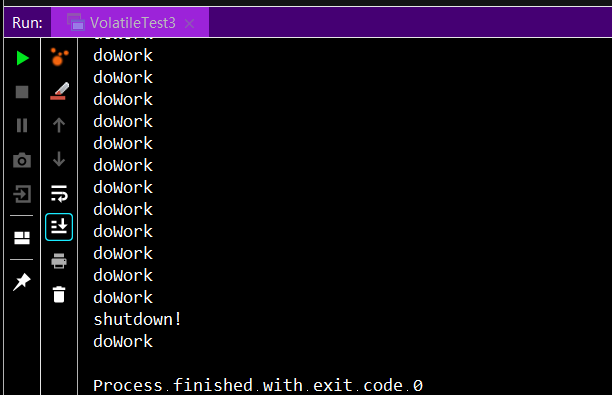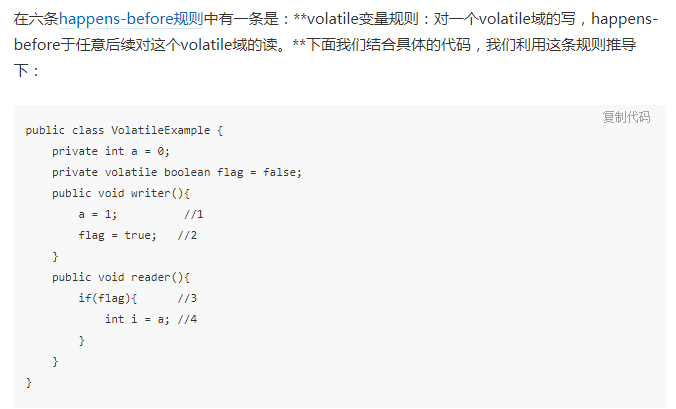# java volatile关键字作用及使用场景详解

更新时间：2019年08月04日 14:18:23   转载 作者：孜然狼

1. volatile关键字的作用：保证了变量的可见性（visibility）。被volatile关键字修饰的变量，如果值发生了变更，其他线程立马可见，避免出现脏读的现象。如以下代码片段，isShutDown被置为true后，doWork方法仍有执行。如用volatile修饰isShutDown变量，可避免此问题。

```public class VolatileTest3 {
static class Work {
boolean isShutDown = false;

void shutdown() {
isShutDown = true;
System.out.println("shutdown!");
}

void doWork() {
while (!isShutDown) {
System.out.println("doWork");
}
}
}

public static void main(String[] args) {
Work work = new Work();

}
}```2. 为什么会出现脏读？

Java内存模型规定所有的变量都是存在主存当中，每个线程都有自己的工作内存。线程对变量的所有操作都必须在工作内存中进行，而不能直接对主存进行操作。并且每个线程不能访问其他线程的工作内存。变量的值何时从线程的工作内存写回主存，无法确定。

3. happens-before规则的理解与勘误```public class VolatileTest {
static class A {
int a = 0;
volatile boolean flag = false;

void writer() {
a = 1;   //1
flag = true;  //2
System.out.println("write");
}

if (flag) {  //3
int i = a;  //4
System.out.println("i is :" + i);
} else {
int i = a;
System.out.println("i is :" + i);
}
}

}

public static void main(String[] args) {
A aaa = new A();
}
}```4. volatile关键字使用场景

```public class VolatileTest2 {
static class A {
volatile int a = 0;
void increase() {
a++;
}
int getA(){
return a;
}
}

public static void main(String[] args) {
A a = new A();

for (int i = 0;i < 1000;i++) {
a.increase();
}
System.out.println(a.getA());
}).start();
for (int i = 0;i < 2000;i++) {
a.increase();
}
System.out.println(a.getA());
}).start();
for (int i = 0;i < 3000;i++) {
a.increase();
}
System.out.println(a.getA());
}).start();
for (int i = 0;i < 4000;i++) {
a.increase();
}
System.out.println(a.getA());
}).start();
for (int i = 0;i < 5000;i++) {
a.increase();
}
System.out.println(a.getA());
}).start();
}
}```volatile正确的使用方法可参考：https://www.jb51.net/article/166888.htm